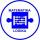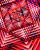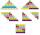# AP - simple

Determine the first nine elements of sequence if a10 = -1 and d = 4

a9 =  -5
a8 =  -9
a7 =  -13
a6 =  -17
a5 =  -21
a4 =  -25
a3 =  -29
a2 =  -33
a1 =  -37

### Step-by-step explanation:

${a}_{9}=-1-4=-5$
${a}_{8}=-5-4=-9$
${a}_{7}=-9-4=-13$
${a}_{6}=-13-4=-17$
${a}_{5}=-17-4=-21$
${a}_{4}=-21-4=-25$
${a}_{3}=-25-4=-29$
${a}_{2}=-29-4=-33$
${a}_{1}=-33-4=-37$Did you find an error or inaccuracy? Feel free to write us. Thank you!## Related math problems and questions:

• AP - simpleFind the first ten members of the sequence if a11 = 132, d = 3.
• Sequence 2Write the first 5 members of an arithmetic sequence a11=-14, d=-1
• AS sequenceIn an arithmetic sequence is given the difference d = -3 and a71 = 455. a) Determine the value of a62 b) Determine the sum of 71 members.
• SequenceWrite the first 7 members of an arithmetic sequence: a1=-3, d=6.
• SequenceWrite the first 6 members of this sequence: a1 = 5 a2 = 7 an+2 = an+1 +2 an
• Sequence 11What is the nth term of this sequence 1, 1/2, 1/3, 1/4, 1/5?
• AS - sequenceWhat are the first ten members of the sequence if a11=22, d=2.
• SequenceIn the arithmetic sequence is a1=-1, d=4. Which member is equal to the number 203?
• SequenceIn the arithmetic sequence is given: Sn=2304, d=2, an=95 Calculate a1 and n.
• GP - sequenceThe first three terms of a geometric sequence are as follows 10, 30. 90, find the next two terms of this sequence.
• Determine APDetermine the difference of the arithmetic progression if a3 = 7, and a4 + a5 = 71
• Finite arithmetic sequenceHow many numbers should be inserted between the numbers 1 and 25 so that all numbers create a finite arithmetic sequence and that the sum of all members of this group is 117?
• Sequence 3Write the first 5 members of an arithmetic sequence: a4=-35, a11=-105.
• SequenceBetween numbers 1 and 53 insert n members of the arithmetic sequence that its sum is 702.
• CoefficientDetermine the coefficient of this sequence: 7.2; 2.4; 0.8
• The sidesThe sides of a right triangle form an arithmetic sequence. The hypotenuse is 24 cm long. Determine the remaining sides of the triangle.
• 6 termsFind the first six terms of the sequence. a1 = 7, an = an-1 + 6